# AP Statistics Practice Test 2

### Test Information10 questions23 minutes

1. Extra study sessions were offered to students after the midterm to help improve their understanding of statistics. Student scores on the midterm and the final exam were recorded. The following scatterplot shows final test scores against the midterm test scores.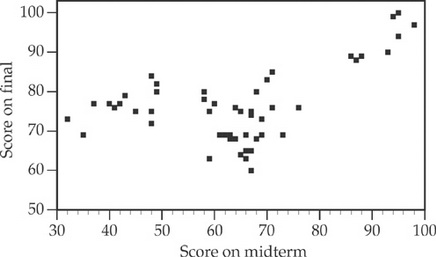Which of the following statements correctly interprets the scatterplot?

2. A resident of Auto Town was interested in finding the cheapest gas prices at nearby gas stations. On randomly selected days over a period of one month, he recorded the gas prices (in dollars) at four gas stations near his house. The boxplots of gas prices are as follows: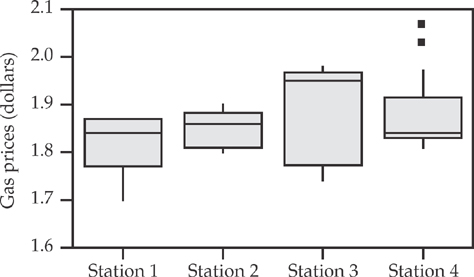Based on this display, which of the stations had the closest mean and median during the month?

3. A botanist is interested in testing H0: μ = 3.5 cm versus Ha: μ > 3.5, where μ = the mean petal length of one variety of flowers. A random sample of 50 petals gives significant results at a 5 percent level of significance. Which of the following statements about the confidence interval to estimate the mean petal length is true?

4. A filling machine puts an average of four ounces of coffee in jars, with a standard deviation of 0.25 ounces. Forty jars filled by this machine are selected at random. What is the probability that the mean amount per jar filled in the sampled jars is less than 3.9 ounces?

5. The number of customers served per day by a large department store is normally distributed, with a mean of 3,250 customers and a standard deviation of 320. Find the range of customers served on the middle 50 percent of days.

6. Assume that the masses of chicken eggs are normally distributed with a mean of 45 g and a standard deviation of 4 g. What mass of egg would be the 25th percentile of the masses of all the eggs?

7. The observed times (in minutes) it takes a runner to complete a marathon are normally distributed. The z-score for his running time this week is –2. Which one of the following statements is a correct interpretation of his z-score?

8. For which of these is a binomial probability model most reasonable?

9. A dentist has noticed that about two children in every seven whom he sees professionally develop cavities before they turn 10 years old. Last week he examined the teeth of five unrelated children younger than 10. Let X be the number of children who develop cavities before turning 10. Which of the following gives the probability that at least one will develop a cavity before turning 10?

10. The probability that Ted enrolls in an English class is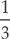. If he does enroll in an English class, the probability that he enrolls in a mathematics class is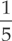. If he does not enroll in an English class, the probability that he enrolls in a mathematics class is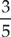. If we learn that Ted enrolled in a mathematics class, what is the probability that he enrolled in an English class?